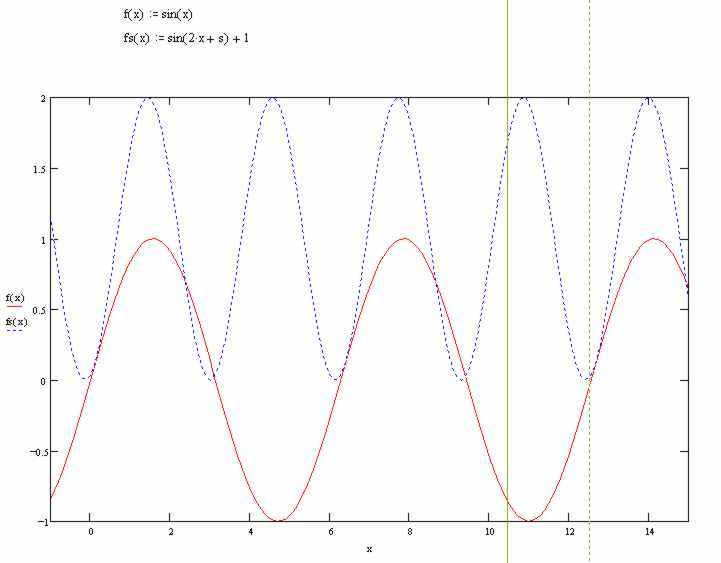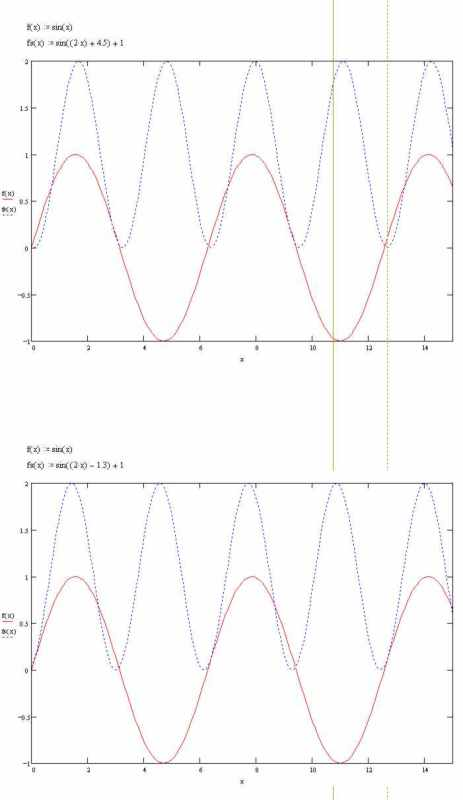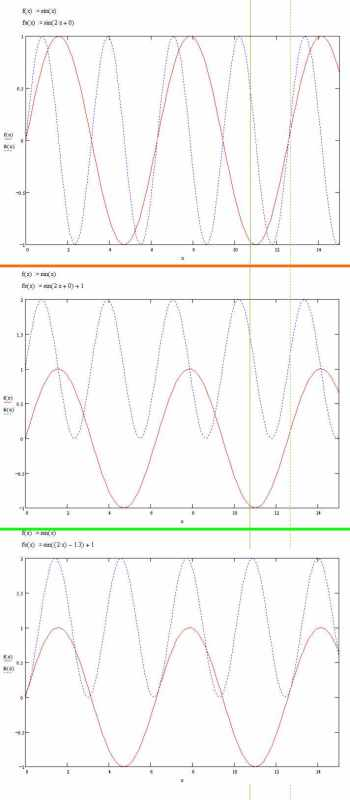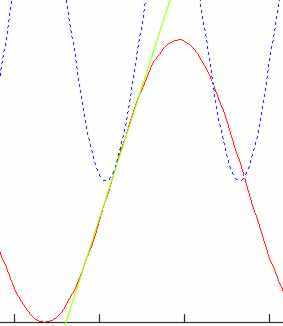×
INTELLIGENT WORK FORUMS
FOR ENGINEERING PROFESSIONALS

Are you an
Engineering professional?
Join Eng-Tips Forums!
• Talk With Other Members
• Be Notified Of Responses
• Keyword Search
Favorite Forums
• Automated Signatures
• Best Of All, It's Free!

*Eng-Tips's functionality depends on members receiving e-mail. By joining you are opting in to receive e-mail.

#### Posting Guidelines

Promoting, selling, recruiting, coursework and thesis posting is forbidden.

# Simultaneous trigonometry equation ?3

## Simultaneous trigonometry equation ?

(OP)
I don't even know what this type of equation is really called, so I'm having difficulty even searching for a solution.

I wonder can anyone show a step by step solution to this, or is it purely a case of solving it by iteration?

sin(x) = sin(2x+s)+1

cos(x) = cos(2x+s)

Find x and s to satisfy the two above statements.

I do believe there are at least two solutions to this. (+ve s and -ve s)

(I suppose in this case there are infinite solutions after each period of sin x, since 2x is a direct multiple of x, but in reality if we suppose 2x was replaced by 1.9278x or some other random value, then the solution is no longer going to be every period of sin x).

The solution has a real life application, but I didn't want to include my application lest it just caused more confusion.

Below is an illustration of a graphically guessed solution. (guessed value of s=1.3)

I'm trying to solve where the two waves are tangential.

Thanks### RE: Simultaneous trigonometry equation ?

(OP)
Thanks, but what's next?

sin(x) = (sin(2x)*cos(s)+sin(s)*cos(2x))+1

cos(x) = cos(2x)*cos(s)-sin(2x)*sin(s)

### RE: Simultaneous trigonometry equation ?

If you're looking for tangent points, it seems like going back to basics is the best path.

If the first derivative of a function gives you the slope of the tangent, you want to determine where the first derivative of one equals the first derivative of the other. The first derivative of each is straightforward, so after that, it's plug and play.

Dan - Owner
http://www.Hi-TecDesigns.com### RE: Simultaneous trigonometry equation ?

(OP)
@MacGyver

Thanks, but that is exactly what I have already done.

The first equation defines possible intersection points, and the second line is the derivatives of each half of the first statement, hence the two tangents are coincident.

### RE: Simultaneous trigonometry equation ?

(OP)
I could also do it iteratively myself, but I want to learn how to do it mathematically rather than just use software, that is if there is a way other than iteration.

### RE: Simultaneous trigonometry equation ?

Start off with something more simple, like s=0. If that's the case, then you have cos(x)=cos(2x). This can only be true when x=0°, right (and 360° increments, if my rusty memory serves)?

So what happens when s!=0?

Dan - Owner
http://www.Hi-TecDesigns.com### RE: Simultaneous trigonometry equation ?

Essentially, they are only equivalent once around the entire unit circle. That point is when x=2x+s, right? So, any time you have s=-x, the equations are equivalent. Solve for any angle x, which gives you a valid value of s. Bob's your uncle.

Dan - Owner
http://www.Hi-TecDesigns.com### RE: Simultaneous trigonometry equation ?

(OP)
Sorry, you totally lost me with that one.

Can you please show it will real numbers to clarify.

Thanks

### RE: Simultaneous trigonometry equation ?

Ignore s for the moment... you're left with cos(x)=cos(2x). The only time that is true over the unit circle is when x=0°, so we only have to worry about one solution, the direct one (no versions of x +/- π/2, etc.).

Bringing s back in, the only way for cos(x)=cos(2x+s) to work is for the "innards" to be equivalent, as well, i.e., x=2x+s. Solving for s, you get s=-x. No matter what value x is, setting s=-x will provide you with an equivalent value on both sides.

Let x=12°. Therefore, s must equal -12° if you want the two sides to be equivalent. When equivalent, the slopes of the signal at that point (x=12°) are also equivalent. Pick another value for x, same thing. If you plot x and s, they are merely negatives of each other(or out of phase by 180°, pick your viewpoint)...

Dan - Owner
http://www.Hi-TecDesigns.com### RE: Simultaneous trigonometry equation ?

(OP)
Well, here are two manually adjusted graphs.

First thing, I was wrong to assume -ve s and +ve s would give the same results.

I had to do a fudge of s = -ve 1.3 or s = +ve 4.5

@MacGuyver You can see in both these solutions that x and s are not a common value of opposite signs.

In the upper solution, when x is about 3, s is 4.5

and in the lower solution, when x is about 0.1, s is -1.3### RE: Simultaneous trigonometry equation ?

(OP)
This is how I have broken it into separate stages, but I still don't know how to calculate the true value of s.

The first stage shows the two superimposed sine waves.

Stage two shifts the higher frequency wave upwards by a value of 1, hence the lowest point is tangential with the horizontal axis.

Stage three then slides the higher frequency wave horizontally until it becomes tangent with the lower frequency wave.

Common in the three plots is sin (x)

Then the superimposed wave is:

Plot one has sin(2x+0)
Plot two has sin(2x+0)+1
Plot three has sin(2x-1.3)+1### RE: Simultaneous trigonometry equation ?

2
https://www.wolframalpha.com/

Enter: Find x and s where sin(x) = sin(2x+s)+1, cos(x) = cos(2x+s)

Disclaimer: I'm not sure if it's interpreted it the way you want or not. Perhaps the input needs adjustment.

### RE: Simultaneous trigonometry equation ?

(OP)
hmmmmm ...

I still can't see any numbers that show s around -1.3 or 4.5 on the Wolfram page

Is there a fundamental flaw in my equation?

Am I correct in defining my first derivatives of

sin(x) and sin(2x+s)+1

as

cos(x) and cos(2x+s) ???

### RE: Simultaneous trigonometry equation ?

I'm not seeing any mistakes in my math (though it's possible they exist)...

In your graph, however, I see crossings, not identical slopes (like at x=3)... not the same thing. It does not appear as if your "solution" is actually one. You cannot look at a graph like that and pick a solution by eye.

You're also graphing the original equations, not their derivatives. If you graph the derivatives, I think you'll find things are a bit different looking...

Dan - Owner
http://www.Hi-TecDesigns.com### RE: Simultaneous trigonometry equation ?

(OP)
@MacGyverS2000 -- But the derivatives are the tangent points.

There's no need to plot them.

They're shown below by the estimated green line, representing the common tangent point.

Thanks for everyone's input, but it appears not such a simple solution after all.

I had hoped someone might be fit to illustrate it if is was very simple, but it seems not to be the case.

Thanks nevertheless.### RE: Simultaneous trigonometry equation ?

The Wolfram Alpha link that I provided above offers an 'Implicit Plot'.

Notice that the Implicit Plot has red dots. If you hover your mouse pointer over the red dots, then numbers will magically appear...

{s= -1.5708, x= 0.523599}
{s= 4.71239, x= 0.523599}
...etc.

Perhaps I'm confused, but those values (presumably truncated) appear to be simultaneous solutions to the OP's original formula (the two formula at the very top of this thread). I checked the first example with the first formula, and it worked out as approximately 0.5 = 0.5. But I can't be bothered to check them all.

The Implicit Plot hints that there are an infinite number of solutions. If I understand the WA output correctly, then the 'Results' formulas provides the machinery to generate the solutions. Crank in the c1 and c2 from 'The Set of all Integers'.

Disclaimer: I'm not very good at math, so hopefully this is a correct interpretation.

### RE: Simultaneous trigonometry equation ?

Bad timing for me. I just tried to solve and was proud tome come up with something. Then realized similar thing was just posted by hacksaw. Oh well.
sin(x) = sin(2x+s)+1
cos(x) = cos(2x+s)

since sin^2 + cos^2 = 1 (lhs), we know we can do the same to the RHS
[sin(2x+s)+1]^2 + cos(2x+s)^2 = 1
[sin^2(2x+s) +2*sin(2x+s)+1] + cos^2(2x+s) = 1

subtract 1 each side
sin^2(2x+s) +2*sin(2x+s) + cos^2(2x+s) = 0

Combine sin^2 and cos^2 into 1
1 + 2*sin(2x+s) = 0
sin(2x+s) = -1/2

=====================================
(2B)+(2B)' ?

### RE: Simultaneous trigonometry equation ?

(OP)
@ hacksaw

surely line 1 of your solution, (as shown below), doesn't reflect what is stated in post #1

You have stated "2Cos" instead of just "Cos"

Sin(x) = 1 + Sin(2x+s)
Cos(x) = 2Cos(2x+s)

### RE: Simultaneous trigonometry equation ?

I don't think he was intending to solve the identical problem you posted, maybe just a similar problem. It points out the use of the simplification sin^2+cos^2=1. That makes the solution pretty simple. I think the way I showed above is the solution unless I made a silly algebra error.

Just to clarify my terminology

#### Quote (electricpete)

since sin^2 + cos^2 = 1 (lhs), we know we can do the same to the RHS
could maybe have been written more succinctly as
LHS(eq1)^2 + LHS(eq2)^2 = 1 ⇒ RHS(eq1)^2 + RHS(eq2)^2 = 1

sin(2x+s) = -1/2
for any given value of x you can generate corresponding values of s as follows:
s = asin(-1/2) - 2x
where
asin(-1/2) = [7*pi/6 + n*(2*pi)] or [11*pi/6 + n*(2*pi)]
and n= any integer positive, negative or zero

=====================================
(2B)+(2B)' ?

### RE: Simultaneous trigonometry equation ?

typical for me, I stand corrected,

### RE: Simultaneous trigonometry equation ?

#### Quote:

sin(x) = sin(2x+s)+1 [eq 1]

cos(x) = cos(2x+s) [eq 2]

Find x and s to satisfy the two above statements.
That's the part I solved above. Call my solution [equation 3]

#### Quote:

I'm trying to solve where the two waves are tangential.
Is that is an additional requirement that needs to be solved?
If so its an additional constraint on the solution... odd to tack it on at the very end and maybe explains why hacksaw was looking at derivatives.
It would still be a somewhat murky requirement (which two out of the four listed functions LHS(1),RHS(1),LHS(2),RHS(2) are supposed to be tangential and in which plane (y,x) or (y,s).

=====================================
(2B)+(2B)' ?

### RE: Simultaneous trigonometry equation ?

(OP)
@electricpete

I don't know what you "mean tack it on at the end"

The fourth line of post #1 provides the derivatives of the two equations in the third line of the first post, so the requirement for the tangential solution was there from the very beginning. See below

I don't even know what this type of equation is really called, so I'm having difficulty even searching for a solution.

I wonder can anyone show a step by step solution to this, or is it purely a case of solving it by iteration?

sin(x) = sin(2x+s)+1

cos(x) = cos(2x+s)
these are the derivatives from the previous line, hence the common tangent definitions..

### RE: Simultaneous trigonometry equation ?

(OP)
So, after all the posts above, I still don't know how to calculate the phase shift.

Possibly it is because I didn't lay out my question correctly in the first instance.

For that reason I decided to reformulate the question and create a new post.

Maybe that will help clarify any confusion.

Thanks

The new post is here

#### Red Flag This Post

Please let us know here why this post is inappropriate. Reasons such as off-topic, duplicates, flames, illegal, vulgar, or students posting their homework.

#### Red Flag Submitted

Thank you for helping keep Eng-Tips Forums free from inappropriate posts.
The Eng-Tips staff will check this out and take appropriate action.

#### Resources

Low-Volume Rapid Injection Molding With 3D Printed Molds
Learn methods and guidelines for using stereolithography (SLA) 3D printed molds in the injection molding process to lower costs and lead time. Discover how this hybrid manufacturing process enables on-demand mold fabrication to quickly produce small batches of thermoplastic parts. Download Now
Examine how the principles of DfAM upend many of the long-standing rules around manufacturability - allowing engineers and designers to place a partâ€™s function at the center of their design considerations. Download Now
Industry Perspective: Education and Metal 3D Printing
Metal 3D printing has rapidly emerged as a key technology in modern design and manufacturing, so itâ€™s critical educational institutions include it in their curricula to avoid leaving students at a disadvantage as they enter the workforce. Download Now

Close Box

# Join Eng-Tips® Today!

Join your peers on the Internet's largest technical engineering professional community.
It's easy to join and it's free.

Here's Why Members Love Eng-Tips Forums:

•Talk To Other Members
• Notification Of Responses To Questions
• Favorite Forums One Click Access
• Keyword Search Of All Posts, And More...

Register now while it's still free!